## Symmetry and the Fourth Dimension (Part 5)

Last time we saw that Platonic solids come in dual pairs, with the tetrahedron being dual to itself:

When you have a dual pair, you can start chopping off the corners of one, more and more, and keep going until you reach the other. Along the way you get some interesting shapes: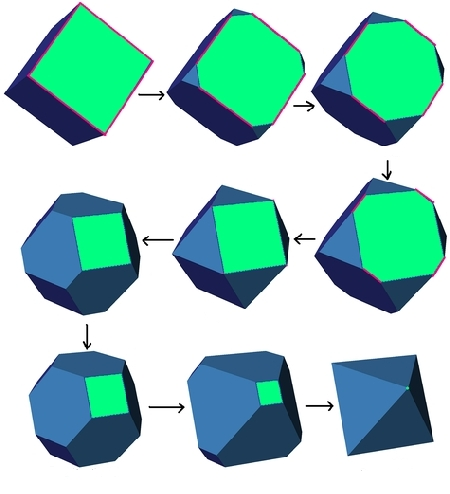At certain points along the way, we get semiregular polyhedra, meaning that:

• all the faces are regular polygons, and

• there’s a symmetry carrying any corner to any other.

Let’s see how it goes with the cube/octahedron pair. And on the way, I’ll show you some diagrams that summarize what’s going on. I’ll explain them later, but you can try to guess the pattern. As a clue, I’ll say they’re based on the Coxeter diagram for the cube, which I explained last time:

V—4—E—3—F

Here we go!

### Cube: •—4—o—3—o

First we have the cube, with all square faces:### Truncated cube: •—4—•—3—o

Then we get the truncated cube, with octagons and triangles as faces:### Cuboctahedron: o—4—•—3—o

Halfway through we get the aptly named cuboctahedron, with squares and triangles as faces:### Truncated octahedron: o—4—•—3—•

Then we get the truncated octahedron, with squares and hexagons as faces:### Octahedron: o—4—o—3—•

Then finally we get the octahedron, with triangles as faces:### Partial flags

Can you see what’s going on with the diagrams here?

 cube•—4—o—3—o truncated cube•—4—•—3—o cuboctahedrono—4—•—3—o truncated octahedrono—4—•—3—• octahedrono—4—o—3—•

Clearly the black dots tend to move from left to right as we move down the chart, but there’s something much cooler and more precise going on. The black dots secretly say where the corners of the shapes are!

Let’s see how quickly I can explain this, and how quickly you can get what I’m talking about. Remember how I defined a ‘flag’ in Part 3?

No? Good, because today I’m going to call that a ‘complete flag’. So, given a Platonic solid, we’ll say a complete flag is a vertex, edge and face where the vertex lies on the edge and the edge lies on the face.

For example, here is a complete flag for a cube: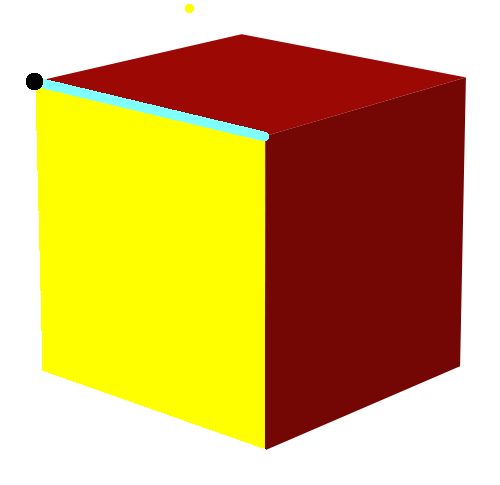It’s the black vertex lying on the blue edge lying on the yellow face.

But the term ‘complete flag’ hints that there are also ‘partial flags’. And there are! A vertex-edge flag is a vertex and edge where the vertex lies on the edge. Here’s a vertex-edge flag for the cube: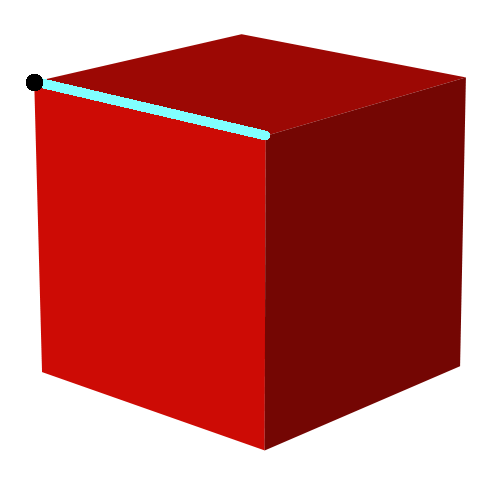Similarly, an edge-face flag is an edge and a face where the edge lies on the face. Here’s an edge-face flag for the cube:You can see why they’re called partial flags: they’re different parts of a complete flag.

Now, fix the Coxeter diagram for the cube firmly in mind:

V—4—E—3—F

V for vertex, E for edge and F for face.

Then:

• a cube obviously has one corner for each vertex of the cube, so we draw it like this:

•—4—o—3—o

• a truncated cube has one corner for each vertex-edge flag of the cube, so we draw it like this:

•—4—•—3—o

• a cuboctahedron has one corner for each edge of the cube, so we draw it like this:

o—4—•—3—o

• a truncated octahedron has one corner for each edge-face flag of the cube, so we draw it like this:

o—4—•—3—•

• an octahedron has one corner for each face of the cube, so we draw it like this:

o—4—o—3—•

Alas, I don’t have the patience to draw all the pictures needed to explain this clearly; I’ll just grab the pictures I can get for free on Wikicommons. Here’s how an octahedron has a corner for each face of the cube: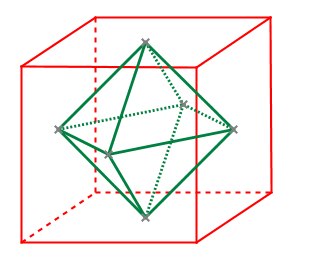And here’s how the cuboctahedron has a corner for each edge of the cube:But these are the least interesting cases! It’s more interesting to see how the truncated cube has one corner for each vertex-edge flag of the cube. Do you see how it works? You have to imagine this truncated cube sitting inside a cube:Then, notice that the truncated cube has 2 corners on each edge of the cube, one near each end. So, it has one corner for each vertex-edge flag of the cube!

Similarly, the truncated octahedron has one corner for each edge-face flag of the cube. But since I don’t have a great picture to help you see that, lets use duality to change our point of view. A face of the cube corresponds to a vertex of the octahedron. So, think of this truncated octahedron as sitting inside an octahedron:The truncated octahedron has 4 corners near each vertex of the octahedron, one on each edge touching that vertex. In short, it has one corner for each vertex-edge flag of the octahedron. So it’s got one corner for each edge-face flag of the cube!

This change of viewpoint can be justified more thoroughly:

Puzzle. The diagrams we’ve been using were based on the Coxeter diagram for the cube. What would they look like if we based them on the Coxeter diagram for the octahedron instead?

By the way, the pretty pictures of solids with brass balls at the vertices were made by Tom Ruen using Robert Webb’s Stella software. They’re available on Wikicommons, and you can find most of them by clicking on the images here and looking around on the Wikipedia articles you’ll reach that way. On this blog, I try hard to make most images take you to more information when you click on them.

### 6 Responses to Symmetry and the Fourth Dimension (Part 5)

1.Greg Egan says:

This is fun, I hadn’t seen this system before!

There’s a tiny typo in the text. The last time you mention the diagram for the octahedron, you repeat the diagram for the truncated octahedron, i.e. you give it as “o—4—•—3—•” rather than “o—4—o—3—•”.

To answer the puzzle as to what the diagrams would look like if they were based on the Coxeter diagram for the octahedron instead of that of the cube, I guess you can just write all the same diagrams backwards.

The Coxeter diagram for the octahedron is:

V—3—E—4—F

So you get:

Cube: o—3—o—4—•

The cube has a vertex for each of the 8 faces of the octahedron.

Truncated cube: o—3—•—4—•

The truncated cube has a vertex for every edge-face flag of the octahedron, so three distinct vertices for each face of the octahedron for a total of 24.

Cuboctahedron: o—3—•—4—o

The cuboctahedron has a vertex for each of the 12 edges of the octahedron.

Truncated octahedron: •—3—•—4—o

The truncated octahedron has a vertex for every vertex-edge flag of the octahedron, so two distinct vertices for each edge for a total of 24.

Octahedron: •—3—o—4—o

And the octahedron is just itself.

•John Baez says:

Great! Thanks for catching that typo—I fixed it.

Yes, if we based our diagrams on the octahedron instead of the cube they wouldn’t change at all!

Okay, well, sure, we’d write them backwards… but that doesn’t really matter: they’ve got enough information in them that we can write them forwards, backwards, or whatever and they still name the same polyhedron.
We should think of them as abstract graphs with edges labelled by numbers and vertices labelled black or white.

Yes, these diagrams are a lot of fun, and the fun is just beginning.

2.Tobias Fritz says:

Cool stuff indeed!

Ok, I understand that the black dots tell us where the vertices of those “intermediate” polytopes are. But what about the edges and the faces of the intermediate polytopes? While those are determined by the vertices, I still wonder: do they also correspond to certain sublattices of the face lattice of the cube? (For example, every edge of the truncated cube corresponds to either an edge of the cube or a diamond in the face lattice of a cube, i.e. two edges meeting in a vertex and spanning a face.)

Is there a nice way to directly read off the whole face lattice of the polytope from its Coxeter diagram equipped with the black markings?

•John Baez says:

Tobias wrote:

Is there a nice way to directly read off the whole face lattice of the polytope from its Coxeter diagram equipped with the black markings?

I’m sure there is, because everything in this subject is maximally nice… and it’s also very well-understood. However, I don’t know how to to it!

I’ll give a clue that may help someone figure this out. I’ve been taking a deliberately lowbrow approach in this series, but there’s a deeper way to understand these labelled Coxeter diagrams which I might or might not get around to explaining in my posts. It goes like this:

A Coxeter diagram like

V—4—E—3—F

can be seen as nothing but a quick way of writing down a group presentation. From the viewpoint, the letters don’t mean anything: they’re just names of the generators. The relations say the generators always square to one:

V2 = E2 = F2 = 1

and then there are the interesting relations, which are described by the diagram:

(VE)4 = 1

(EF)3 = 1

(VF)2 = 1

where last has a 2 in the exponent because there’s no edge joining V and F–that’s just one of the rules.

Let’s call this group G. In this outlook, a labelled Coxeter diagram is a way of naming a subgroup H. The vertices of the corresponding polyhedron (or higher-dimensional polytope) are the points of G/H.

I think the way I have it set up, the subgroup H is generated by the white vertices. So, for example, if we take this labelling:

•—4—o—3—o

we get a subgroup H that’s isomorphic to

E—3—F

But this diagram describes the symmetry group of an equilateral triangle. You can see this directly from the presentation encoded by the diagram E—3—F, namely:

E2 = F2 = 1

(EF)3 = 1

So in this case H is the symmetry group of an equilateral triangle, with 6 elements. So, since G has 48 elements, G/H has 48/6 = 8 elements, which sounds right for the vertices of the cube.

But it’s not just that we’re getting the right number! If you look at our operation E that changes which edge of the cube a chosen triangle touches: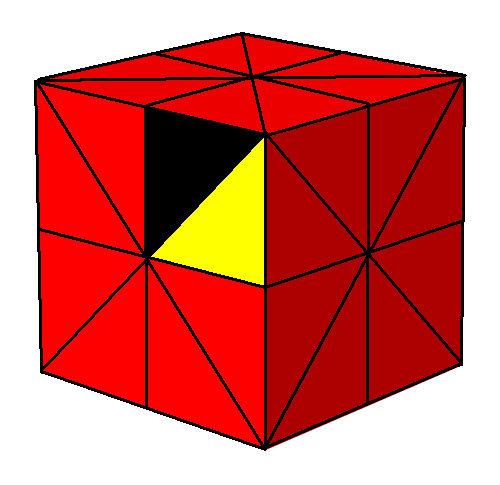and the operation F that changes which face of the cube that triangle lies on: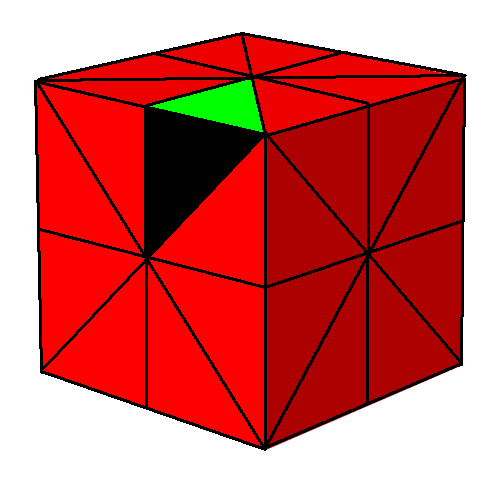you’ll see they both fix the same vertex of the cube: the vertex closest to us! And indeed, H is the group of all symmetries of the cube that fix that vertex—the so-called ‘stabilizer’ of the vertex. So, by some famous abstract nonsense, G/H can be seen as the set of vertices of the cube.